# ISEE Lower Level Math : Data Analysis and Probability

## Example Questions

### Example Question #1 : How To Use A Venn Diagram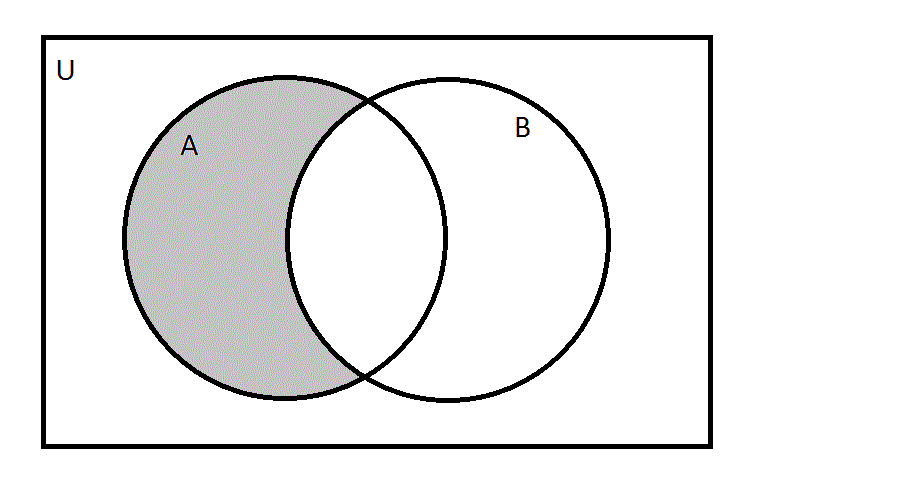Define universal set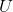to be the set of integers from 1 to 20 inclusive.

Define sets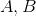as follows: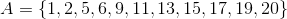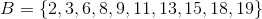Which of the following would be the set of all elements that would go into the grayed region in the above Venn diagram?

Possible Answers: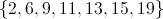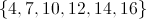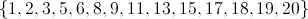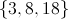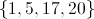Correct answer:Explanation:

The grayed region is the set of all elements that are in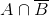- that is, inbut not. This set is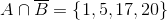### Example Question #1 : How To Use A Venn Diagram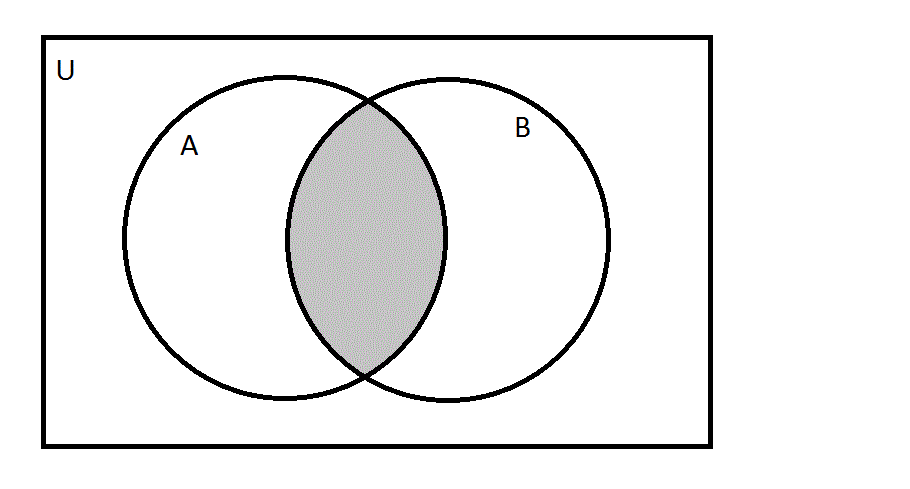Define universal setto be the set of integers from 1 to 20 inclusive.

Define setsas follows:Which of the following would be the set of all elements that would go into the grayed region in the above Venn diagram?

Possible Answers:Correct answer:Explanation:

The grayed region is the set of all elements that are in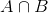- that is, in bothand. This set is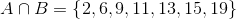### Example Question #101 : Data Analysis And Probability

The following Venn diagram depicts the number of students who like soccer, football, or both. How many students like soccer but not football?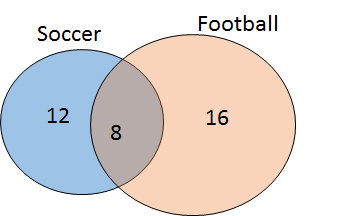Possible Answers:

8

16

12

28

Correct answer:

12

Explanation:

A Venn diagram is used to demonstrate the number of people (or things) in particular categories. The regions of the circle that are overlapping indicate the number of individuals that belong to multiple groups. Therefore, based on this diagram, 12 students like soccer only, 16 like football only, and 8 like both.

### Example Question #1 : How To Use A Venn Diagram

Use the Venn Diagram to answer the question.

All of the sixth grade students at Park Middle School play at least one instrument.There are 60 total sixth graders at Park Middle School.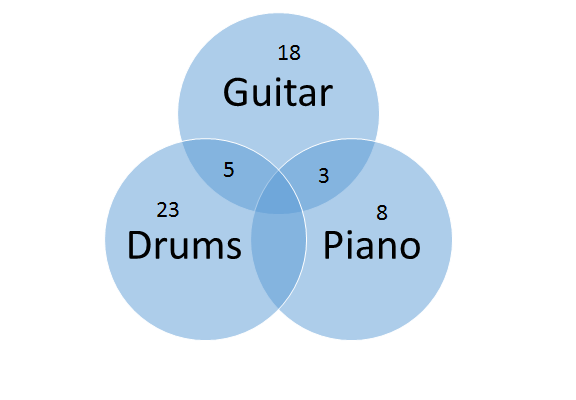How many students play both the drums and the guitar, but do not play the piano?

Possible Answers: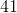Correct answer:Explanation:

The shaded area where the circle that contains the word guitar overlaps with the circle that contains the word drums has the number 5.  It is incorrect to add the total number of guitar players to the total number of drum players because we are only looking for students who play both instruments.

### Example Question #101 : Data Analysis And Probability

Use the Venn Diagram to answer the question.

All of the sixth grade students at Park Middle School play at least one instrument.There are 60 total sixth graders at Park Middle School.Based on the information in the diagram, how many students play the guitar?

Possible Answers:Correct answer:Explanation:

18 students play the guitar, but you must also add the 5 students who play the guitar and drums and the 3 students who play the guitar and piano.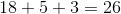### Example Question #101 : Data Analysis And Probability

Use the Venn Diagram to answer the question.

All of the sixth grade students at Park Middle School play at least one instrument.There are 60 total sixth graders at Park Middle School.If one student plays all three instruments, how many students play both the drums and the piano, but not the guitar?

Possible Answers:Correct answer:Explanation:

There are 60 total students. If 1 person plays all 3 instruments, then add that to all of the other numbers on the Venn Diagram to see that  58 students are represented on the Venn Diagram. Subtract that number from the total number of students to find the number of students in the missing category (those who play both the drums and the piano, but not the guitar).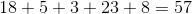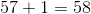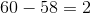### Example Question #6 : How To Use A Venn Diagram

The intersection point of a venn diagram represents the probability of niether event happening.  True or false?

Possible Answers:

False

True

Correct answer:

False

Explanation:

The intersection of a venn diagram represents when both events happen at the same time, not when neither happen.

### Example Question #7 : How To Use A Venn Diagram

Use the following Venn Diagram to answer the question.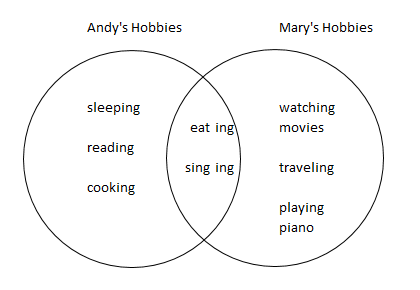What hobbies does Andy enjoy doing?

Possible Answers: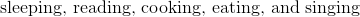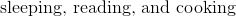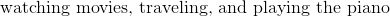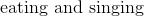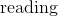Correct answer:Explanation:

When looking at a Venn Diagram, we can see that it is made up of two circles.  In this Venn Diagramwe can see Andy's hobbies on the left and Mary's hobbies on the right.  We also see what hobbies they have in common in the center where the two circles intersect.

Now, when looking at Andy's circle containing his hobbies, we see that he enjoys sleeping, reading, and cooking.  His circle is also part of the intersection, so we must include those hobbies as well.  So, he also enjoys eating and singing.

So, overall, Andy's hobbies include sleeping, reading, cooking, eating, and singing.

### Example Question #8 : How To Use A Venn Diagram

The area when two circles on a Venn Diagram cross each other is when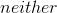of the events occurs.  True or False?

Possible Answers: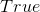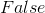Correct answer:Explanation:

The point where two circles on a Venn Diagram intersect is the point where both of the events occur, not when neither occur.

### Example Question #1 : How To Use A Venn Diagram

Use the following Venn Diagram to answer the question.What hobbies does Andy enjoy doing?

Possible Answers:Correct answer:Explanation:When looking at a Venn Diagram, we can see that it is made up of two circles.  In this Venn Diagram we can see Andy's hobbies on the left and Mary's hobbies on the right.  We also see what hobbies they have in common in the center where the two circles intersect.

Now, when looking at Andy's circle containing his hobbies, we see that he enjoys sleeping, reading, and cooking.  His circle is also part of the intersection, so we must include those hobbies as well.  So, he also enjoys eating and singing.

So, overall, Andy's hobbies include sleeping, reading, cooking, eating, and singing.

### All ISEE Lower Level Math Resources